Reach Us+44-1522-440391
Explicit Calculations of Tensor Product Coefficients for E7 | OMICS International
Journal of Generalized Lie Theory and Applications
All submissions of the EM system will be redirected to Online Manuscript Submission System. Authors are requested to submit articles directly to Online Manuscript Submission System of respective journal.

# Explicit Calculations of Tensor Product Coefficients for E7

Gungormez M* and Karadayi HR

Department of Physics, Faculty of Sciences and Letters, Istanbul Technical University, Maslak, 34469 Istanbul, Turkey

*Corresponding Author:
Gungormez M
Department of Physics, Faculty of Sciences and Letters
Istanbul Technical University, Maslak
34469 Istanbul, Turkey
Tel: +90 212 2853220
Fax: +90 212 2856386
E-mail: [email protected]

Received Date: October 28, 2016; Accepted Date: February 17, 2017; Published Date: February 27, 2017

Citation: Gungormez M, Karadayi HR (2017) Explicit Calculations of Tensor Product Coefficients for E7. J Generalized Lie Theory Appl 11: 254. doi: 10.4172/1736- 4337.1000254

Copyright: © 2017 Gungormez M, et al. This is an open-access article distributed under the terms of the Creative Commons Attribution License, which permits unrestricted use, distribution, and reproduction in any medium, provided the original author and source are credited.

Visit for more related articles at Journal of Generalized Lie Theory and Applications

#### Abstract

We propose a new method to calculate coupling coefficients of E7 tensor products. Our method is based on explicit use of E7 characters in the definition of a tensor product. When applying Weyl character formula for E7 Lie algebra, one needs to make sums over 2903040 elements of E7 Weyl group. To implement such enormous sums, we show we have a way which makes their calculations possible. This will be accomplished by decomposing an E7 character into 72 participating A7 characters.

#### Keywords

Coupling coefficients; Lie algebra; Irreducible representations; Subdominants; Tensor coupling coefficients

#### Introduction

Let G7=E7,A7 and Λ, Λ′ be two dominant weights of G7 where R(Λ) and R(Λ′) are corresponding irreducible representations. For general terms, we follow the book of Humphreys  as ever.

Tensor product of these two irreducible representations is defined by,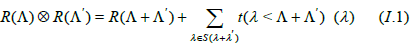where S(λ+λ′) is the set of Λ+Λ′ subdominants and t(λ<Λ+Λ′) s are tensor coupling coefficients. Though Steinberg formula is the best known way, a natural way to calculate tensor coupling coefficients is also to solve the equation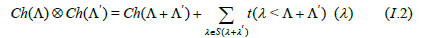for tensor coupling coefficients. Ch(λ) here is the character of an irreducible representation R(λ) which corresponds to a dominant weight λ and it is defined by the famous Weyl Character formula: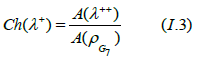where for a weight μ in general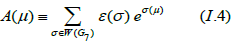W(G7) is the Weyl Group of G7 and each and every element σ is the so-called Weyl reflection while ε(σ) denotes its sign and eσ (λ ++ ) ’s here are known as formal exponentials.Thoroughout this work, we assume λ++ λ ++ denotes a strictly dominant weight defined for a dominant λ+ by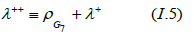where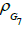is the Weyl vector of G7.

The crucial fact here is that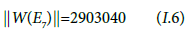where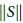denotes order of set S. It is easy to see then to implement the sum in (I.4) would not be realizable explicitly. We, instead, propose 72 specifically chosen Weyl reflections which give us A7 dominant weights participating within the same E7 Weyl orbit W(Λ+) for any E7 dominant weight Λ+. As it is shown in the next section, this makes the evaluation of (I.4) realizable for E7 but in terms of 72 A7 characters and hence easily implementable.

#### A7 Decomposition of E7 Lie Algebra

For i=1,2…,7, let λi’s and αi’s be respectively the fundamental dominant weigths and simple roots of A7 Lie algebra with the following Dynkin diagram (Figure 1).

where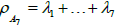is A7 Weyl vector and Λ i’ s be fundamental dominant weights of E7 Lie algebra in according with the following Dynkin diagram, (Figure 2).

where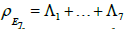is A7 Weyl vector. We suggest following relations allows us to embed A7 subalgebra into E7 algebra: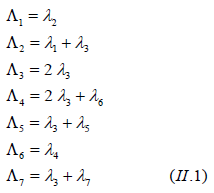This essentially means that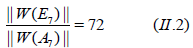which tells us that there are at most 72 A7 dominant weights inside a Weyl orbit W(Λ+). Note here that it is exactly 72 when Λ+ is a strictly dominant weight. From the now on, W(μ) will always denotes the Weyl orbit of a weight μ.

As the main point of view of this work, we present in appendix, 72 Weyl reflections to give 72 A7 dominant weights participating in the same E7 Weyl orbit W(Λ+) when they are exerted on the dominant weight Λ+. To this end, the Weyl reflections with respect to simple roots αi will be called simple reflections σi. We extend multiple products of simple reflections trivially by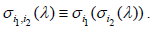For s=1,…72, Σ(s)’s are 72 Weyl reflections mentioned above. As will also be seen by their definitions that,

1) ε(σ(s))=+1 s=1,2,…,36

2) ε(σ(s))=−1 s=37,38,…,72

#### Calculating Tensor Coupling Coefficients

Let us proceed in the instructive example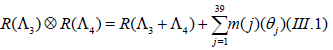of (I.1). One can see that there are 39 sub-dominant weights θj of Λ34 :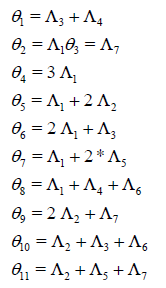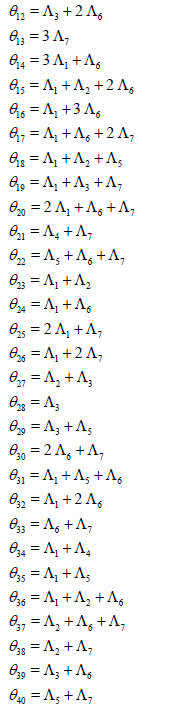To this end, we should care about specialization of formal exponentials . Let us consider the so-called Fundamental Weights μI which are defined for (I=1,…8) as in the following :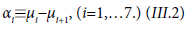αi’s here are A7 simple roots mentioned above and the best way to calculate A7 and hence E7 characters is to use the specialization in terms of parameters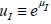which are subjects of the condition μ12+… +μ8=0 or

u1u2… u8=1.

To exemplify (I.3) for E7, we would like to give detailed calculation of Ch(Λ34). By applying 72 specifically chosen Weyl reflections on strictly dominant weight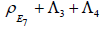, one can see we have the following decompositions: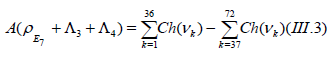where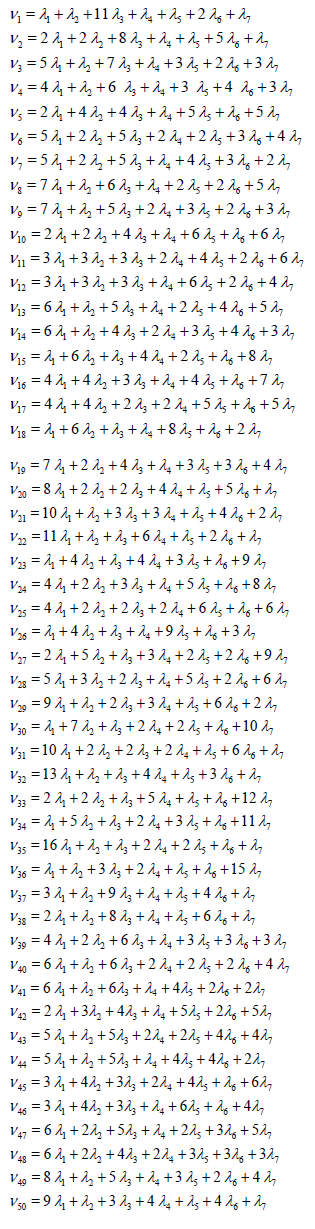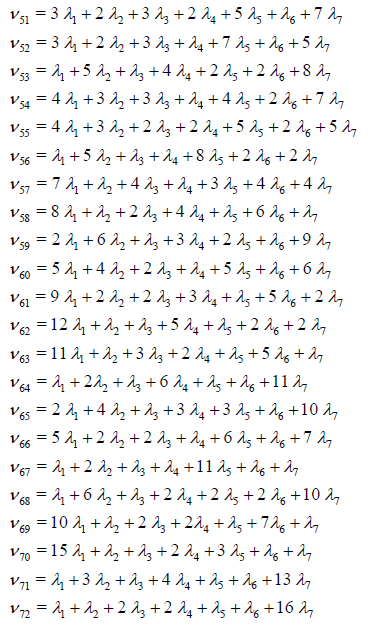A7 characters Ch(vk) ’s are defined by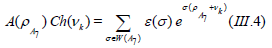Note here that W(A7) is the permutation group of 8 objects.

To display our result here, we use the following specialization of formal exponentials with only one free parameter x: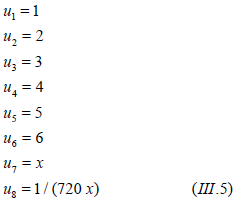In this specialization, one obtains the following one-parameter characters: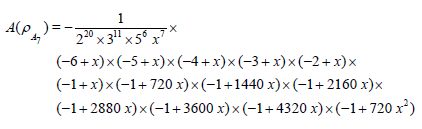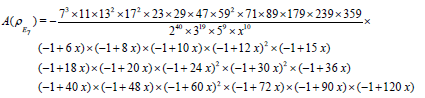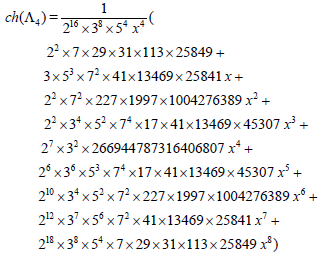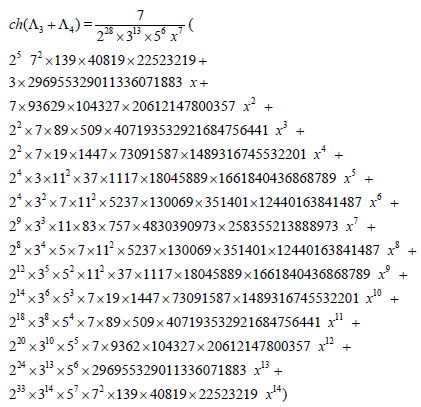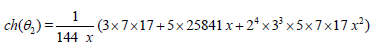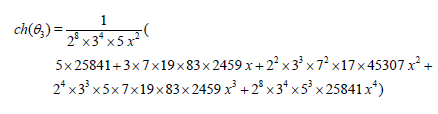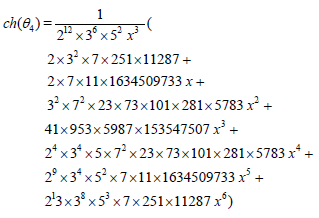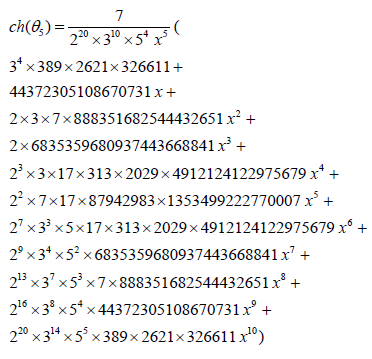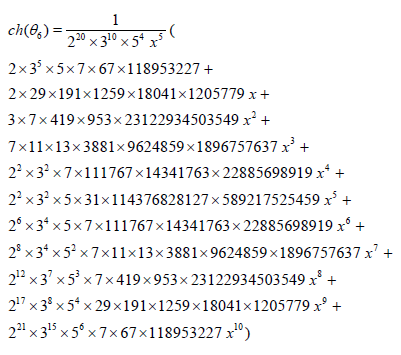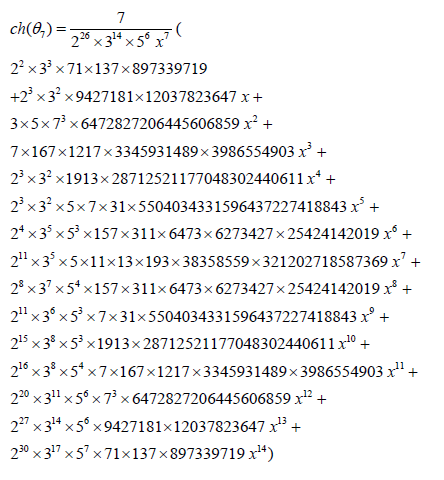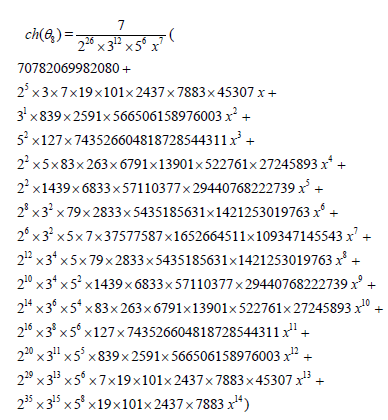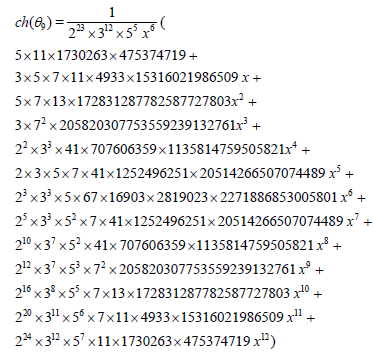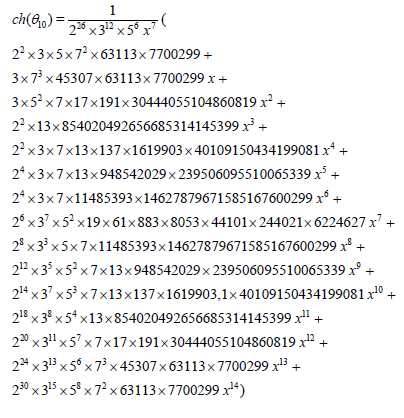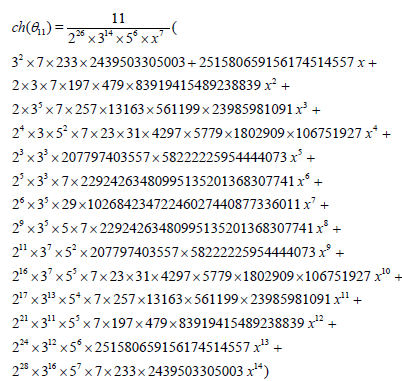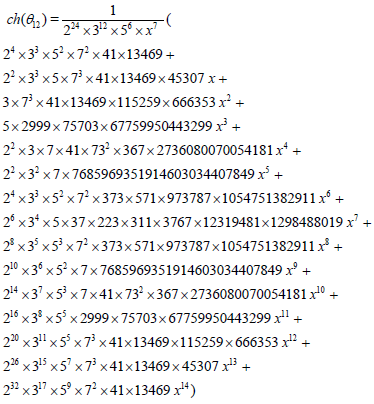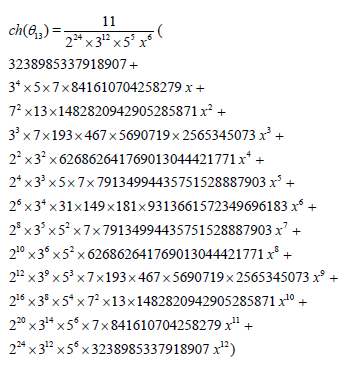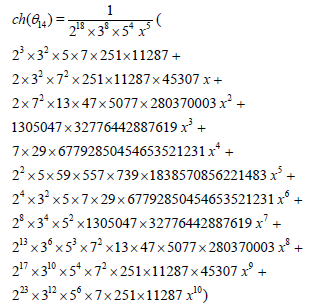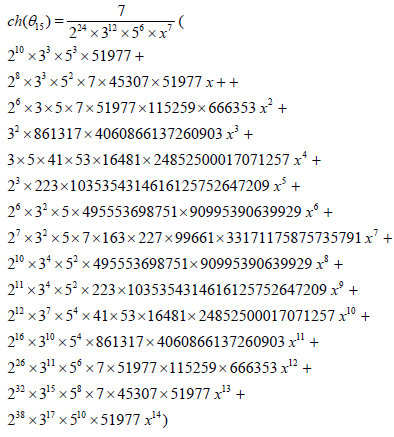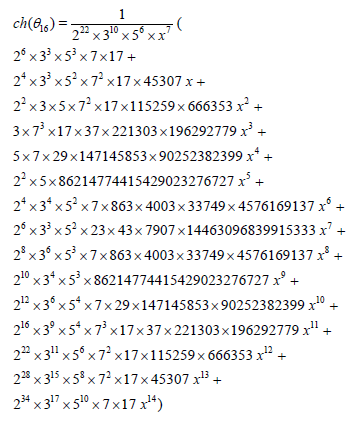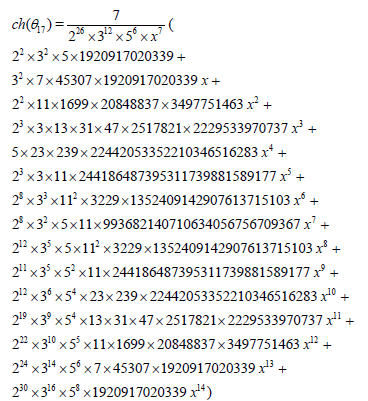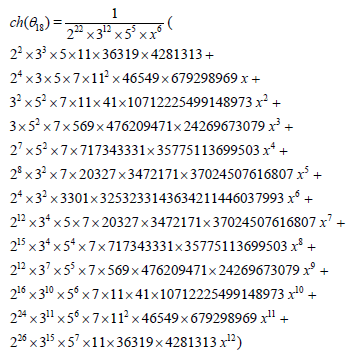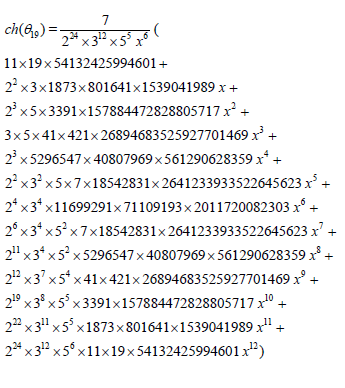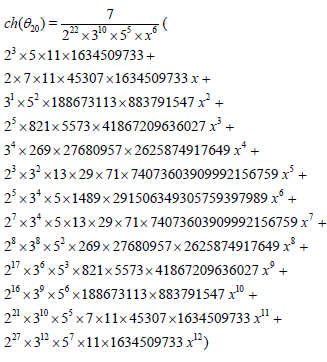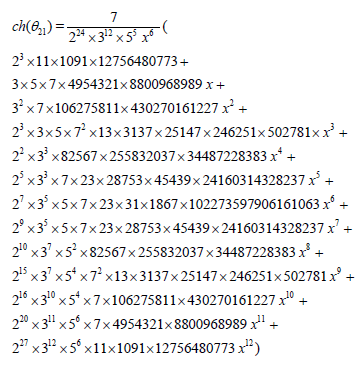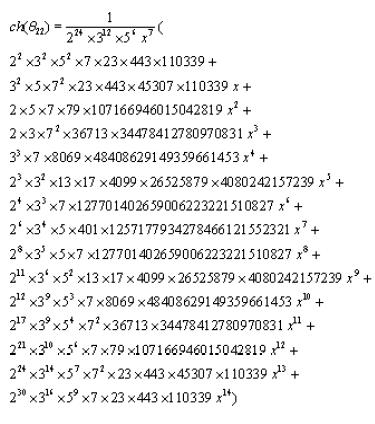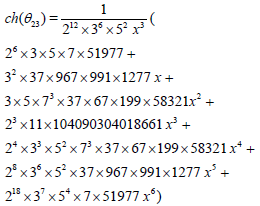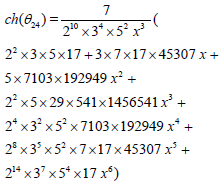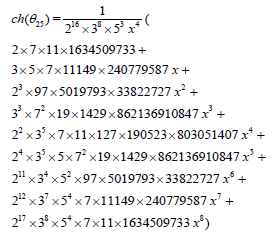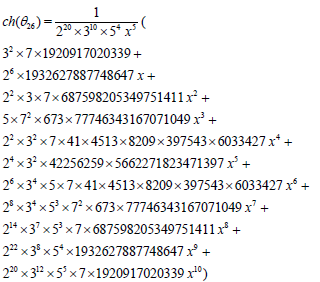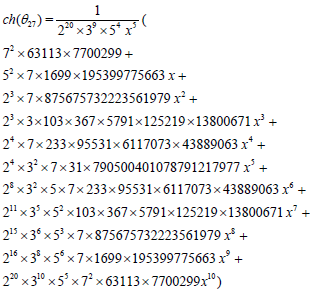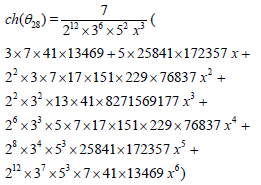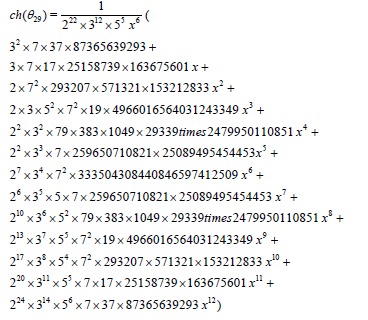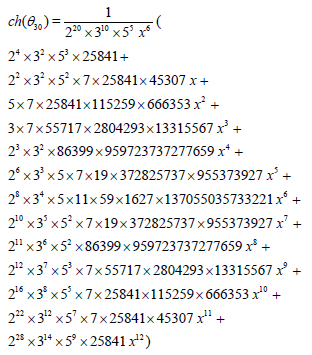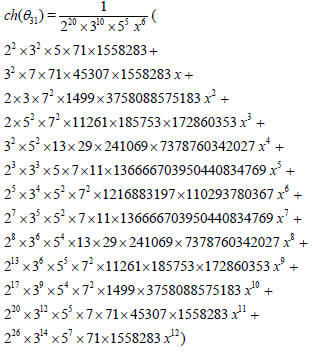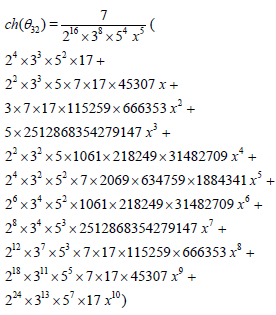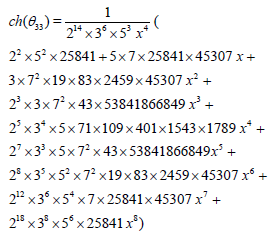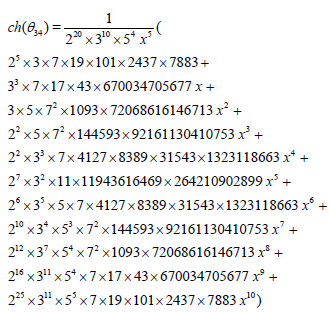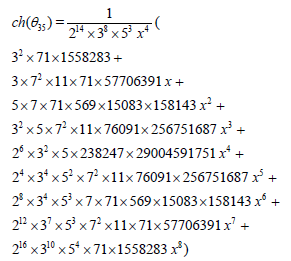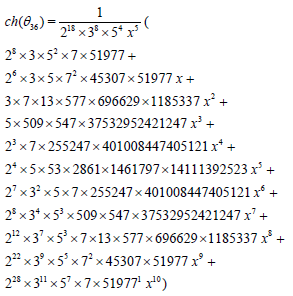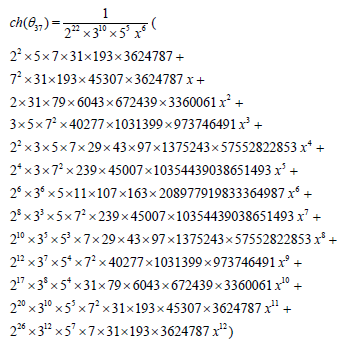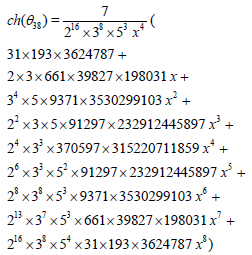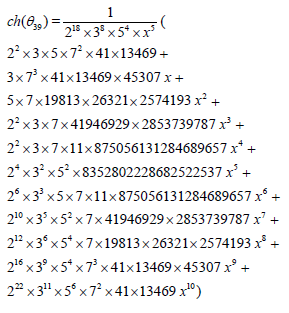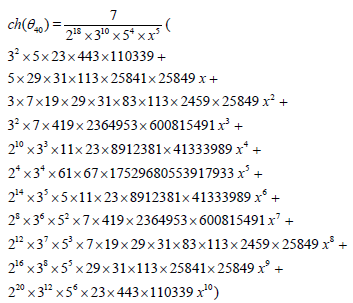Now, one can see that the characters above fulfill the following equation: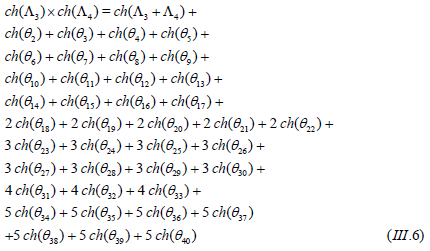#### Conclusion

One should note however that, the 1-parameter specialization (III.5) above is not enough to find all the tensor coupling coefficients completely so we saw that at least 3-parameters specializations will be sufficient, which we used the following one.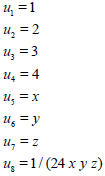#### References

Select your language of interest to view the total content in your interested language

### Article Usage

• Total views: 1125
• [From(publication date):
April-2017 - May 22, 2019]
• Breakdown by view type
• HTML page views : 1041
• PDF downloads : 84

## Post your commentCan't read the image? click here to refresh
###### Peer Reviewed Journals

Make the best use of Scientific Research and information from our 700 + peer reviewed, Open Access Journals

International Conferences 2019-20

Meet Inspiring Speakers and Experts at our 3000+ Global Annual Meetings

Top